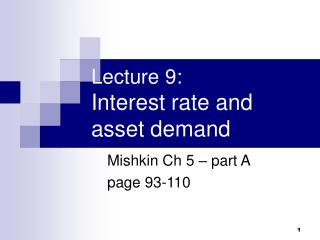DownloadDownload PresentationLecture 9: Interest rate and asset demand

# Lecture 9: Interest rate and asset demand

Télécharger la présentation## Lecture 9: Interest rate and asset demand

- - - - - - - - - - - - - - - - - - - - - - - - - - - E N D - - - - - - - - - - - - - - - - - - - - - - - - - - -
##### Presentation Transcript

1. Lecture 9: Interest rate and asset demand Mishkin Ch 5 – part A page 93-110

2. How is interest rate determined • determined in the bond market • by forces of demand and supply (D&S) • equals market equilibrium • procedure: draw D&S curves, find equilibrium, see what happens if D&S curves shift.

3. Shape of supply and demand ( D&S ) curves • At lower prices (higher interest rates), ceteris paribus, the quantity demanded of bonds is higher - an inverse relationship  slope down • At lower prices (higher interest rates), ceteris paribus, the quantity supplied of bonds is lower - a positive relationship  slope upwards

4. Draw supply and demand curves • X-axis: quantities • Y-axis: prices (of bond) • demand curve slope downwards • supply curve slope upwards • intersection is the equilibrium – market price of a bond, which implies interest rate for the bond.

5. Movements along the curves

6. Market equilibrium • Occurs when the amount that people are willing to buy (demand) equals the amount that people are willing to sell (supply) at a given price. • When Bd = Bs  the equilibrium (or market clearing) price and interest rate. • When Bd > Bs  excess demand  price will rise and interest rate will fall. • When Bd < Bs  excess supply  price will fall and interest rate will rise.

7. Will the equilibrium change? • Would change if there are demand-side or supply-side ‘shocks’ - D&S curves shift.

8. Demand-side (buy-side) factors • Wealth—the total resources owned by the individual, including real assets and financial assets. • Expected Return—the return expected over the next period on one asset relative to alternative assets. • Risk—the degree of uncertainty associated with the return on one asset relative to alternative assets. • Liquidity—the ease and speed with which an asset can be turned into cash relative to alternative assets.

9. How do they affect demand? Holding all other factors constant, the quantity demanded of an asset is • positively related to wealth • positively related to its expected return relative to alternative asset • negatively related to the risk of its returns relative to alternative assets • positively related to its liquidity relative to alternative assets

10. Shifts of the demand curve • Wealth—in an expansion with growing wealth, the demand curve for bonds shifts to the right • Expected Returns—higher expected interest rates in the future lower the expected return for long-term bonds, shifting the demand curve to the left • Expected Inflation—an increase in the expected rate of inflations lowers the expected return for bonds, causing the demand curve to shift to the left • Risk—an increase in the riskiness of bonds causes the demand curve to shift to the left • Liquidity—increased liquidity of bonds results in the demand curve shifting right

11. Shift of the demand curve

12. Supply-side (sell-side) factors • Expected profitability of investment opportunities - in an expansion, the supply curve shifts to the right. • Expected inflation - an increase in expected inflation shifts the supply curve for bonds to the right. • Government budget - increased budget deficits shift the supply curve to the right

13. Why interest rate change? • Demand side factors change • Supply side factors change • Demand side and supply side factors combined • Market equilibrium change

14. Example: expected inflation

15. Example: business expansion

16. Recap • Theory of asset demand • demand and supply curves in bond market • changes in some factors that would affect demand and supply • shifts in demand and supply curves  changes equilibrium bond price (interest rate). • 2 examples